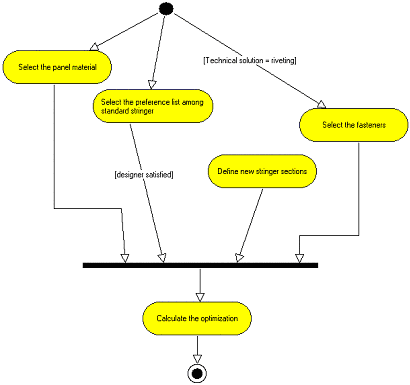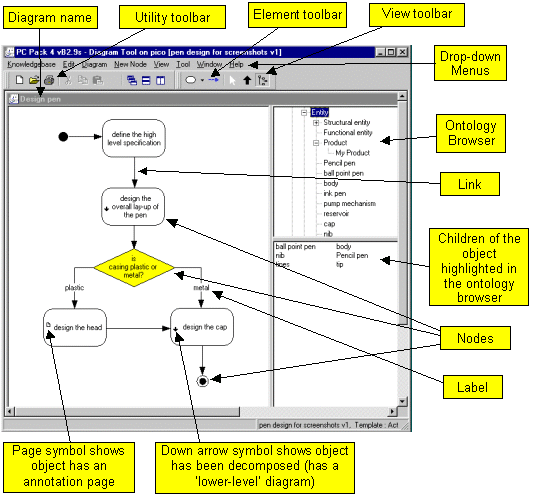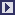## PCPACK6 Diagram Tool

 The Diagram Tool is used to create and edit diagrams. Most types of network-style diagrams can be represented, i.e. those with nodes and links. Nodes and Links Nodes in a diagram can be of two types: (1) those that represent knowledge objects in the knowledgebase, (2) those that are purely for diagrammatic purposes (such as start and stop symbols on some forms of process map). Each link on a diagram between nodes of the first type represents a relationship between the two objects in the knowledgebase (as is the case between two nodes in a ladder). The types of nodes and links used in a diagram are determined by a diagram template (created using the Diagram Template Tool). Decomposing Nodes A particular important feature of the Diagram Tool is the way a hierarchy of tasks can be built and displayed. This is achieved by using a kind of 3-dimensional feel, whereby double-clicking on a node (that has been set-up for decomposition) will reveal a lower-level diagram representing that knowledge object in more detail. In this way, one can start by modelling a knowledge object (e.g. a task) at a very high level (made up of a few sub-tasks), then double-click on each of these knowledge objects (e.g. sub-tasks) to build the next layer of the hierarchy. This provides representations that are much clearer to understand than having a single, large 2-dimensional diagram, and allows the user to set the scale of what knowledge is being captured and modelled. Basic Features Any number of diagrams Any type of diagram format Diagram templates Pre-set (e.g. UML, MOKA, SPEDE) Customisable Designed from scratch Multi-level diagram structures (fully consistent with the relevant ladder) Drag-and-drop existing nodes ontology browser Example Diagram Three examples of the kinds of diagrams that can be created using the Diagram Tool are shown below.In the example shown above, the nodes are special UML-style nodes. Within these, the class of the node has been automatically inserted (shown in angled brackets in the example above). In addition, the properties (attributes and values) of the node have been automatically inserted (shown in the lower box of each node in the example above). User Interface The user interface of the Diagram Tool is explained in the following figure.Other PCPACK tools:Protocol ToolLadder ToolMatrix ToolAnnotation ToolAdmin ToolPublisher ToolDiagram Template ToolEquation Editor ToolLauncher Wizard Tool